# 1. 什么是NeRF

NeRF 是 2020 年 ECCV 上获得最佳论文荣誉提名的工作，其影响力是十分巨大的，不论是后续的学术论文还是商业落地，都引起了大量从业人员的关注。 NeRF 将隐式表达推上了一个新的高度，仅用 2D 的 posed images 作为监督，即可表示复杂的三维场景，在新视角合成这一任务上的表现是非常 impressive 的。当然 NeRF 在其他领域也逐渐开始展露头角，作为新兴的方向，势必也会和传统方法发生碰撞融合，产生新的火花。在B站中有比较详细的课程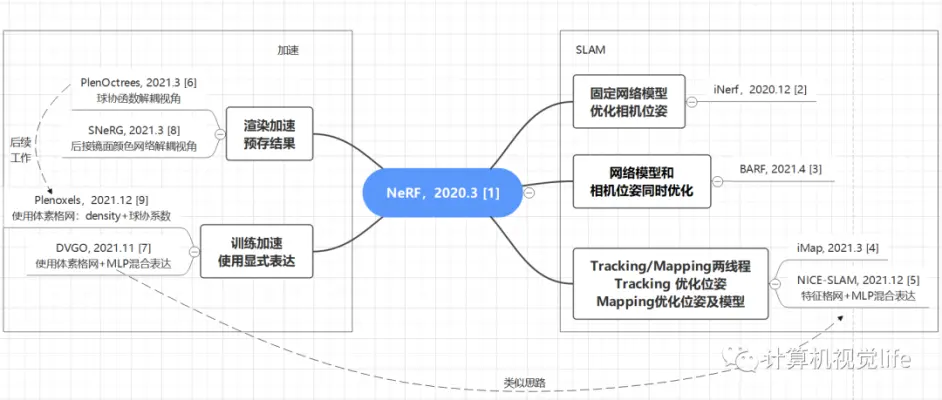我们先来熟悉一下NeRF具体含义。简单的来说，NeRF工作的过程可以分成两部分：三维重建和渲染。

1. 三维重建部分本质上是一个2D到3D的建模过程，利用3D点的位置（ $x,y,z$ ）及方位视角（ $θ,φ$ ）作为输入，通过多层感知机（MLP）建模该点对应的颜色color（ $c$ ）及体素密度volume density（ $σ$ ），形成了3D场景的”隐式表示“。• 体积密度（不透明度） σ 只与三维位置 $x,y,z$ 有关而与视角方向 d 无关。物体不同位置的密度应该和观察角度无关.可以理解为该像素体无论从哪个角度观察，其本身性质是不变的。
• 颜色 c 与三维位置 $x,y,z$ 和视角方向 $θ,φ$ 都相关。由于光照等影响，各个角度观察同一个东西肯定颜色不同。

“显示表示”：3D场景包括Mesh，Point Cloud，Voxel，Volume等，它能够对场景进行显式建模，但是因为其是离散表示的，导致了不够精细化会造成重叠等伪影，更重要的是，它存储的三维场景表达信息数据量极大，对内存的消耗限制了高分辨率场景的应用。 ”隐式表示“：3D场景通常用一个函数来描述场景几何，可以理解为将复杂的三维场景表达信息存储在函数的参数中。因为往往是学习一种3D场景的描述函数，因此在表达大分辨率场景的时候它的参数量相对于“显示表示”是较少的，并且”隐式表示“函数是种连续化的表达，对于场景的表达会更为精细。

1. 渲染部分本质上是一个3D到2D的建模过程，渲染部分利用重建部分得到的3D点的颜色及不透明度沿着光线进行整合得到最终的2D图像像素值。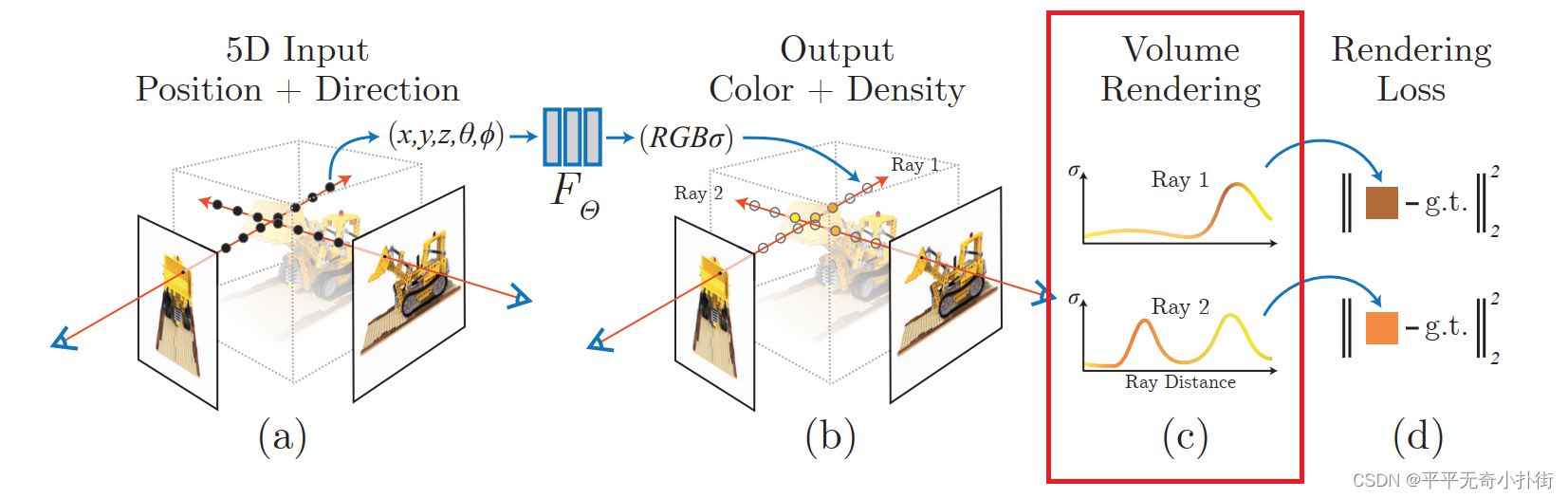这部分最重要的就是：可见光的颜色 RGB 就是不同频率的光辐射作用于相机的结果。因此在 NeRF 中认为辐射场就是对于颜色的近似建模。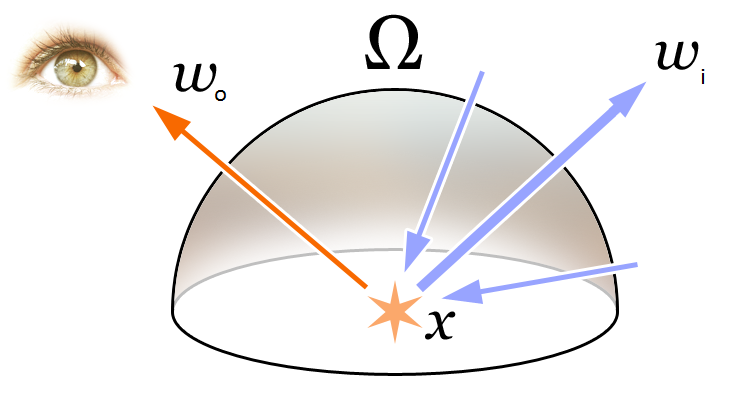$C(r)=\int_{t_n}^{t_f}T(t)σ(r(t))c(r(t), d)dt,whereT(t)=\int_{t_n}^{t}σ(r(s))ds$ 上面的是式子包含了我们第一部分提到的 $σ(r(t))$ $c(r(t), d)$ ，其中函数T(t)表示射线从tn到t沿射线累积透射率，即射线从tn到t不碰到任何粒子的概率； $r(t)=o+td$ ，具体含义为视角 $o$ 发出的方向为 $t$ 时刻到达点,表达的是位置信息。

$\hat{C}(r)=\sum_{i=1}NT_i(1-exp(-\sigma_i\delta_i))c_i,whereT_i=exp(-\sum_{j=1}^{i-1}\sigma_j\delta_j)$

1. 在训练的时候，利用渲染部分得到的2D图像，通过与Ground Truth做L2损失函数（L2 Loss）进行网络优化。（即下图的红框部分）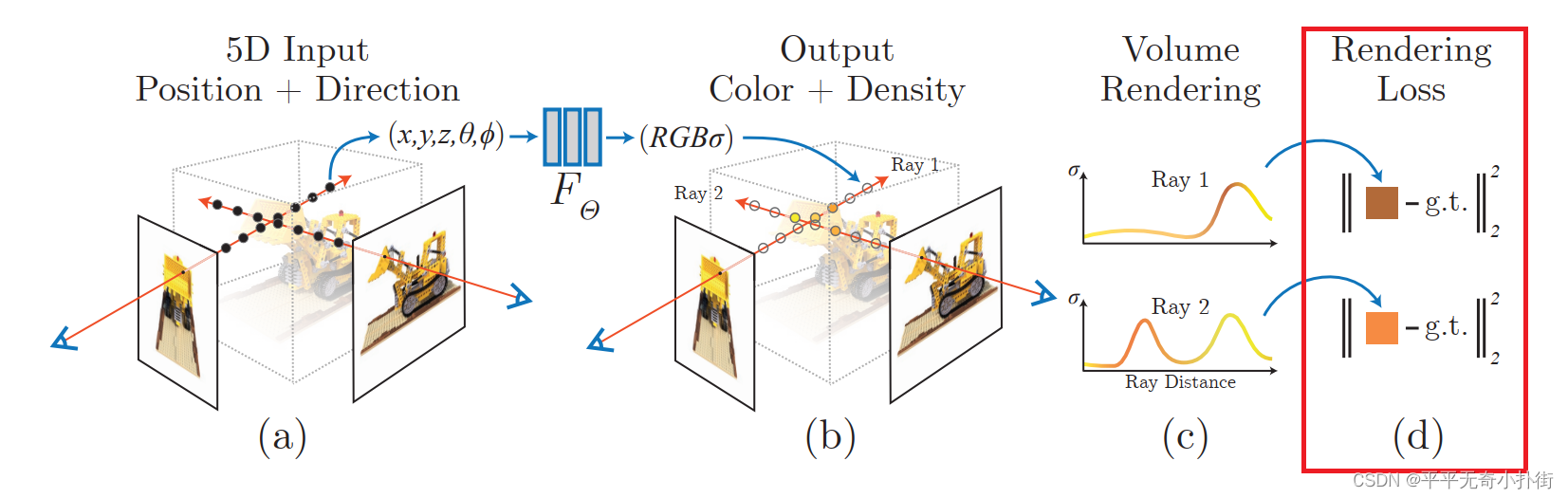总体来说就是通过输入位姿以及2维图像（用于计算损失），得到3D的体素和不同方向的RGB。但是仍然存在训练细节不够精细，渲染在表示颜色和几何形状方面的高频变化方面表现不佳，训练速度慢等原因，为了进一步提升重建的精度和速度，引入了如下两个策略：
• 位置编码(Positional Encoding）:使得MLP学习到的函数nerf能更好的表示高频信息，使用高频函数将输入映射到更高维度的空间，可以更好地拟合包含高频变化的数据。该高频编码函数为： $\gamma(p)=(sin(2^0\pi p),cos(2^0\pi p),…,sin(2^{L-1}\pi p),cos(2^{L-1}\pi p))$
• 金字塔采样方案(Hierarchical Sampling Procedure)：该部分指出在Volume Rendering中是在每条相机光线上的N个查询点密集地评估神经辐射场网络，这是低效的（仍然重复采样与渲染图像无关的自由空间和遮挡区域），于是提出一种分层体积采样的做法，同时优化一个“粗糙”的网络和一个“精细”的网络。通过这一策略，能使训练过程更高效地采样高频信息，该方法对于颜色贡献大的点附近采样密集，贡献小的点附近采样稀疏，从而由粗到细的分层采样方案。

$Loss=\sum_{r\in R}[||\hat C_c(t)-C(r)||_2^2+||\hat C_f(t)-C(r)||_2^2]$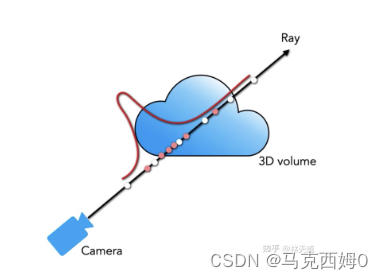# 3.参考链接

https://blog.csdn.net/u013019296/article/details/128572889

https://blog.csdn.net/MAX_Hope/article/details/128253888